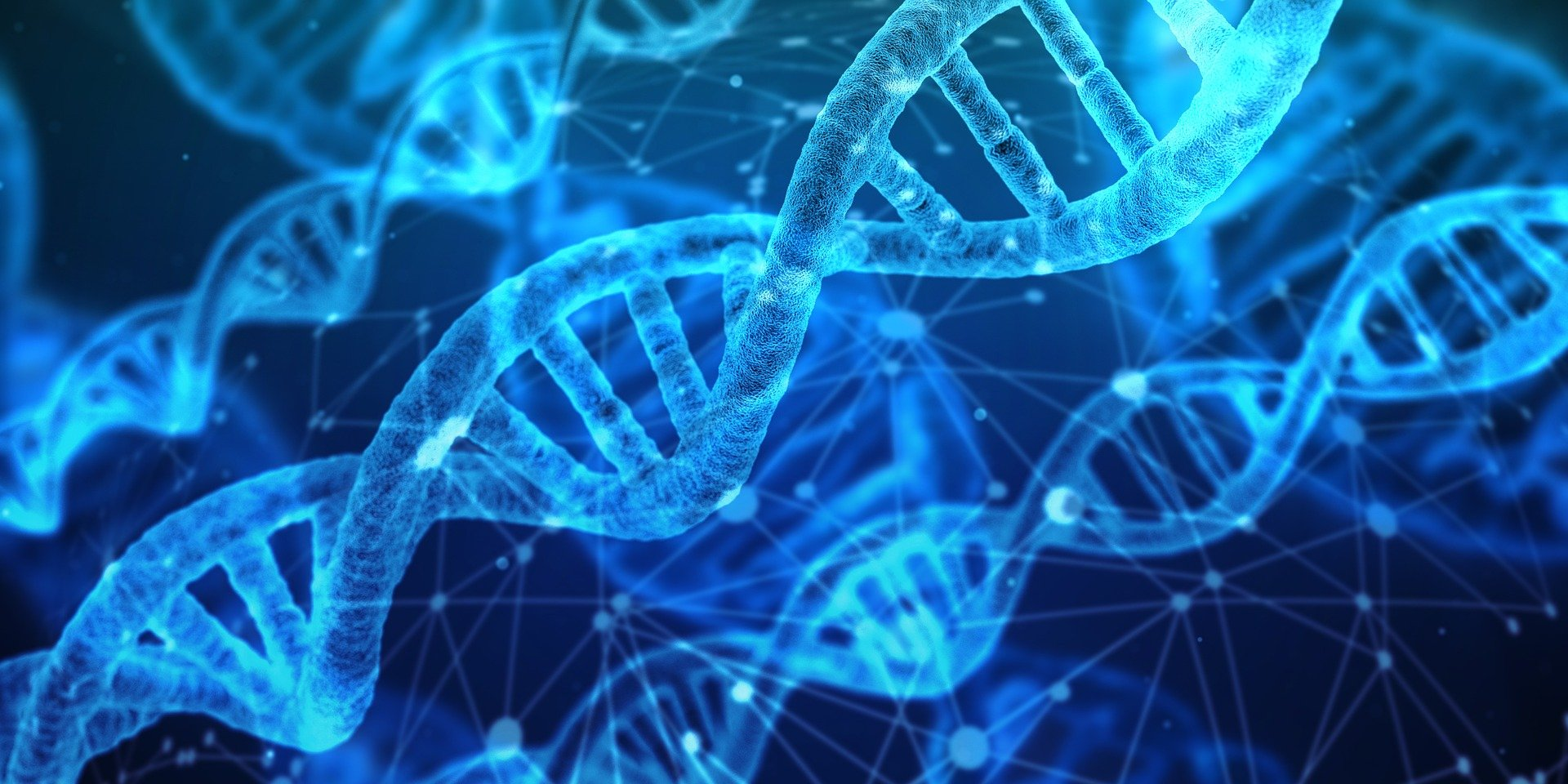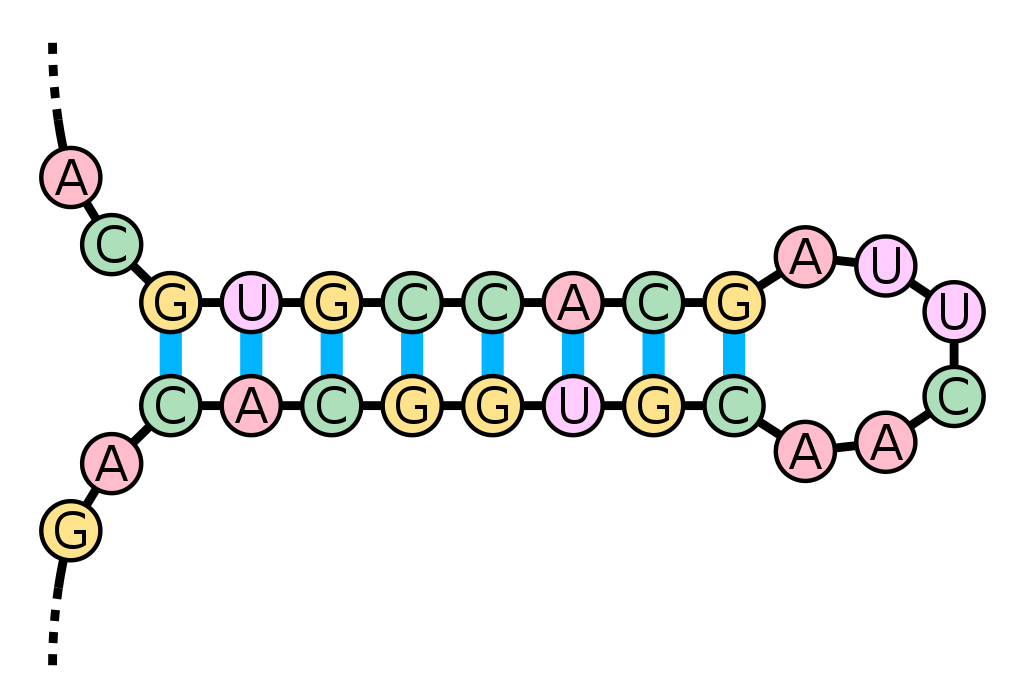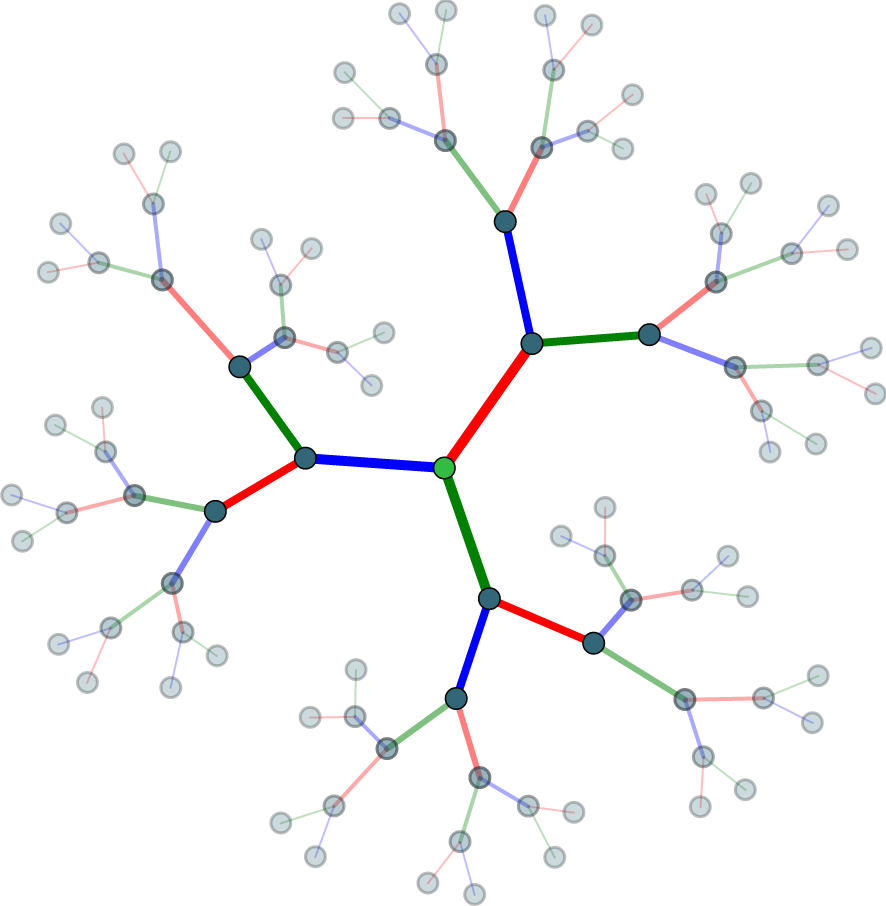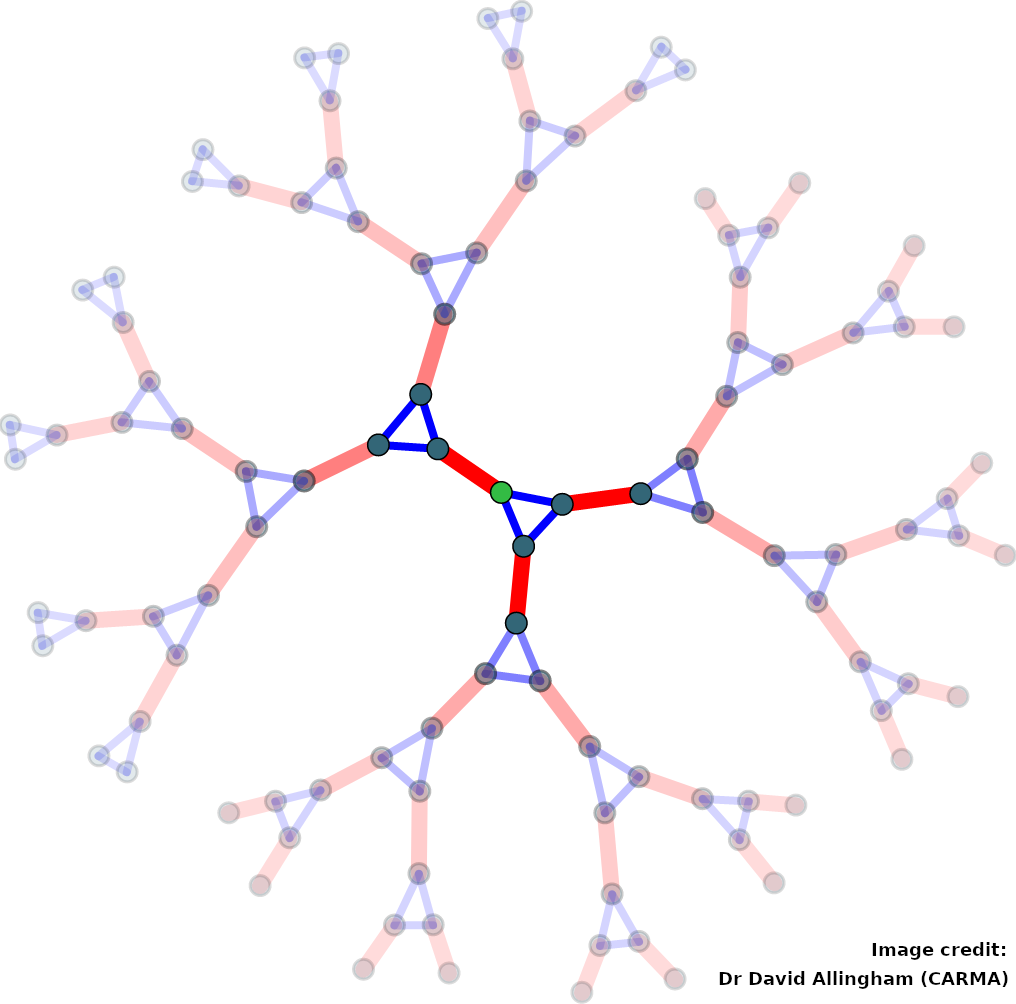# Research Group — Zero-Dimensional Symmetry

## National Youth Science Forum

### The Year 12 Program of the National Youth Science offers students an exciting range of in-person and digital science, technology, engineering, and mathematics (STEM) experiences. For example, at The University of Newcastle, students had the opportunity of "Discovering Symmetry" in a workshop run by Stephan Tornier.## Ph.D. scholarship available

### A Ph.D. position is available in 2021 to work with Dr Stephan Tornier and other members of the Zero-Dimensional Symmetry Research Group on the ARC DECRA project "Effective classification of closed vertex-transitive groups acting on trees".

Candidates with a strong interest and demonstrated skills in at least one of the following areas are encouraged to apply: (topological) group theory, computational algebra (e.g. with GAP), graph theory, combinatorics.

## DECRA success

### Stephan Tornier has been awarded a Discovery Early Career Researcher Award for his project "Effective classification of closed vertex-transitive groups acting on trees". The award funds his position for three years, a Ph.D. scholarship and research cost.## Summer Projects 2020/21

### The following student research projects are related to the Australian Research Council project on 0-Dimensional Symmetry. While they are independent, each one gives a different view on the overall project. Another, more technical, description of this research is that it concerns totally disconnected, locally compact groups. An overview which attempts to explain the broader research project to non-experts may be seen here. (MFO, CC BY-NC-SA 3.0 )

 Isolated points in colourings of vertex transitive graphs (Michal Ferov) The aim of this project is to describe infinite symmetric graphs that admit a rigid colouring. In the case of locally finite graph, the space of all minimal colourings can be naturally seen as a topological space. This project is concerted with the question of to what extent does the topology of the space of colourings correspond to the geometry and symmetries of the graphs. First natural step is to study how far is the space of colourings away from being perfect, i.e. studying the isolated points. In the space of colourings, isolated points correspond to colourings that are rigid, meaning that if any other colouring agrees with it on a large enough finite subgraph, then they have to be the same. The goal of the project is to give some combinatorial of graphs that graphs that admit rigid colourings, or the converse - a combinatorial description of graphs that do not admit rigid colourings. The project is part of a program of research on 0-dimensional groups, which includes symmetries of infinite graphs. Students working on this project will further their knowledge of combinatorics and graph theory. Knowledge of point-set topology and group theory is advantageous but not necessary. Local actions of algebraic groups acting on trees (Stephan Tornier) Symmetry is a fundamental organising principle in mathematics, science and and the arts. It is formalised in the algebraic notion of a 'group'. The symmetry groups of infinite networks, or graphs, constitute a current research frontier. It has proven fruitful to study these groups by analysing their 'local actions', i. e. the permutation groups that the fixator of a vertex in the graph induces on spheres of varying radii around that vertex. The primary aim of this project is to make the local actions of several theoretically derived symmetry groups of graphs tractable by implementing them on a computer using computational group theory tools, such as GAP. A second step would be to study the resulting permutation groups and their interdependence using a mix of theoretical and computational tools. A student who takes this project will extend his/her knowledge of algebra and learn how to use computer algebra systems designed for computations in group theory, including coding skills. Geometric representations of self-replicating groups (George Willis) This project aims to find geometries that have so-called self-replicating, or fractal, groups as their symmetries. The self-replicating nature of these groups is described by representing them as symmetries of rooted trees but that way of thinking about them hides other patterns that are of interest. The purpose of the project is to see the groups geometrically. Computer algebra software will be used to analyse how the groups act on pairs, triples, etc. of vertices of the trees and then study the polyhedra in which these are edges, faces, etc. Observed patterns in the geometries may be extrapolated to produce new families of self-replicating groups. The project is part of a program of research on symmetry groups of infinite networks, known as 0-dimensional groups. Self-replicating 0-dimensional groups are analogous to eigenvalues and eigenvectors in linear algebra and its applications. It is not necessary to understand this bigger picture in order to do the project however. Students taking this project will extend their knowledge of algebra, graph theory and mathematical software. 'Group' is an algebraic notion and 'rooted tree' is a combinatorial one which arises in the study of data structures. Symmetry of Graphs (George Willis) A graph (a network of vertices and edges) is \emph{vertex-transitive} if it 'looks' the same at all vertices. In algebraic terms, this means that, for any pair of vertices, there is an automorphism of the graph which maps the first vertex to the second. A necessary, but not sufficient, condition for a graph to be vertex-transitive is that all vertices should have the same valency. Analysing graph symmetry therefore involves a deeper study of the relationship between graphs and their automorphism groups than vertex valencies. Vertex-transitive graphs need not be edge-transitive, for example, the horizontal edges of a triangular pyramid lie on 3-cycles in the graph but the vertical edges do not. The particular question investigated in this project is how its symmetry group changes as edges are added to, or removed from, a graph. For finite graphs, a classical theorem of Burnside is relevant when the graph has prime order and, for infinite graphs, the goal is to reduce the vertex valency since this number controls important features of the symmetry group. Students taking this project will extend their knowledge of combinatorics and algebra, and how these two topics interact. The project will be jointly supervised by Brian Alspach. Visualising $0$-dimensional symmetry (George Willis) The word 'symmetry' brings to mind visual images and geometry. It has a broader meaning in mathematics, where we think of regularly repeating patterns and invariance under transformations as displaying symmetry, and where the language of algebra is used to describe symmetry. Visualising the patterns or the dynamics of the transformations remains an effective tool for understanding the algebra however. This project aims to develop software for visualising various aspects of $0$-dimensional symmetry, which is the symmetry of infinite networks and arises in number theory and other parts of algebra as well. The aim is to produce software which may be used by researchers and which will be made available on web-pages of the $0$-Dimensional Symmetry project. Students taking this project will extend their knowledge of algebra, analysis, mathematical software and coding skills. 'Totally disconnected' and 'locally compact' are topological notions; 'group' is an algebraic one; and other concepts will be met in the course of the project.